Publicité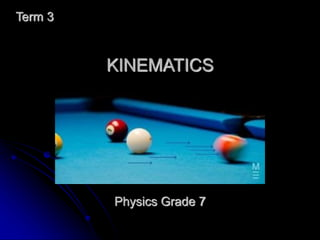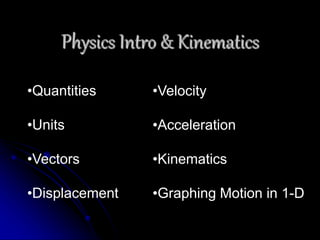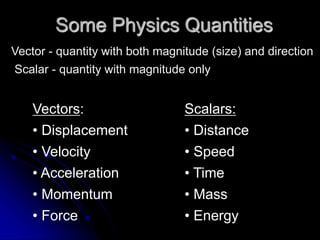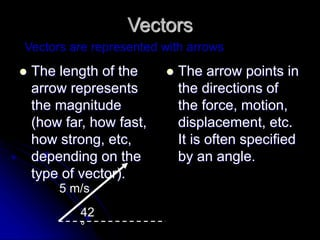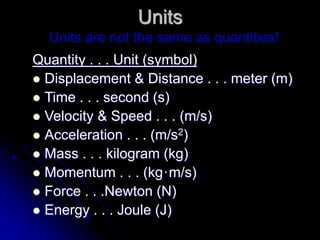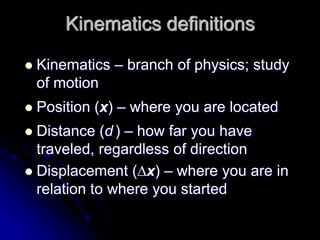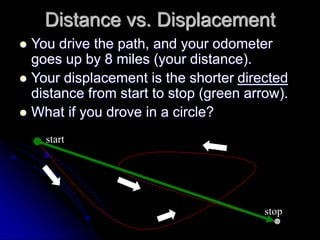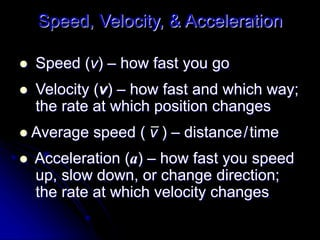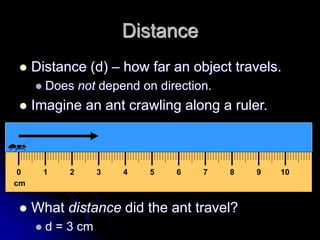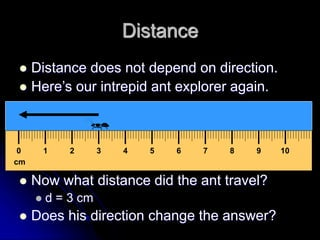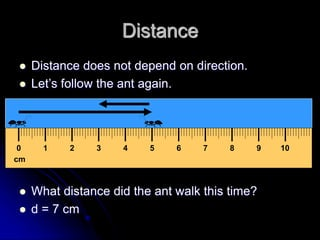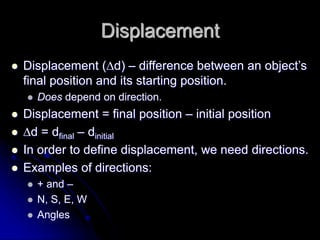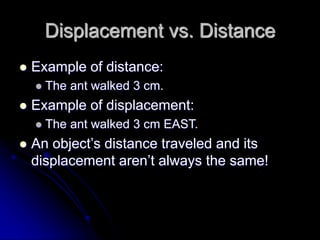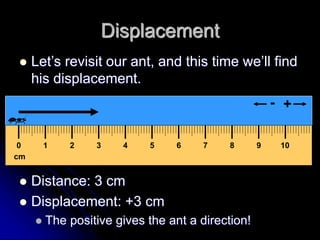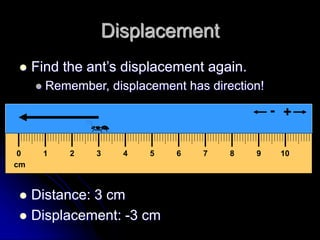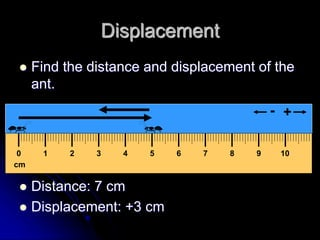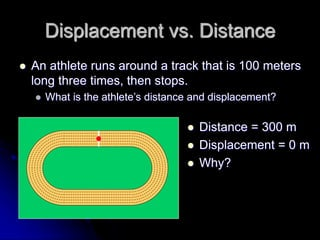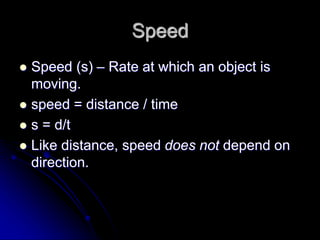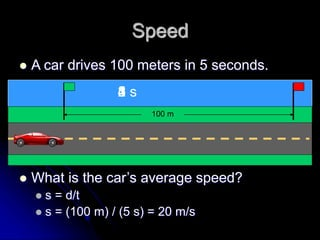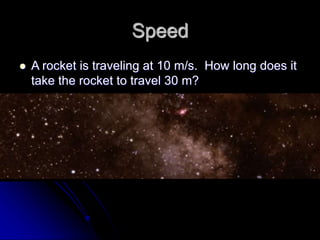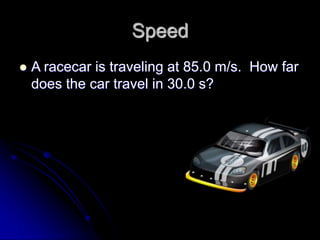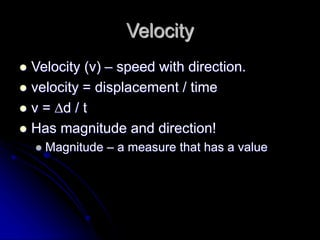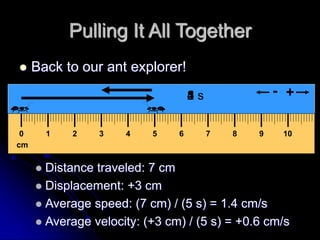1 sur 23
Publicité

### Term 3-Chapter 1-Material 1-Kinematics.ppt

1. KINEMATICS Term 3 Physics Grade 7
2. Physics Intro & Kinematics •Quantities •Units •Vectors •Displacement •Velocity •Acceleration •Kinematics •Graphing Motion in 1-D
3. Some Physics Quantities Vector - quantity with both magnitude (size) and direction Scalar - quantity with magnitude only Vectors: • Displacement • Velocity • Acceleration • Momentum • Force Scalars: • Distance • Speed • Time • Mass • Energy
4. Vectors  The length of the arrow represents the magnitude (how far, how fast, how strong, etc, depending on the type of vector).  The arrow points in the directions of the force, motion, displacement, etc. It is often specified by an angle. Vectors are represented with arrows 42 ° 5 m/s
5. Units Quantity . . . Unit (symbol)  Displacement & Distance . . . meter (m)  Time . . . second (s)  Velocity & Speed . . . (m/s)  Acceleration . . . (m/s2)  Mass . . . kilogram (kg)  Momentum . . . (kg·m/s)  Force . . .Newton (N)  Energy . . . Joule (J) Units are not the same as quantities!
6. Kinematics definitions  Kinematics – branch of physics; study of motion  Position (x) – where you are located  Distance (d ) – how far you have traveled, regardless of direction  Displacement (x) – where you are in relation to where you started
7. Distance vs. Displacement  You drive the path, and your odometer goes up by 8 miles (your distance).  Your displacement is the shorter directed distance from start to stop (green arrow).  What if you drove in a circle? start stop
8. Speed, Velocity, & Acceleration  Speed (v) – how fast you go  Velocity (v) – how fast and which way; the rate at which position changes  Average speed ( v ) – distance/time  Acceleration (a) – how fast you speed up, slow down, or change direction; the rate at which velocity changes
9. Distance  Distance (d) – how far an object travels.  Does not depend on direction.  Imagine an ant crawling along a ruler.  What distance did the ant travel?  d = 3 cm cm 0 1 2 3 4 5 6 7 8 9 10
10. Distance  Distance does not depend on direction.  Here’s our intrepid ant explorer again.  Now what distance did the ant travel?  d = 3 cm  Does his direction change the answer? cm 0 1 2 3 4 5 6 7 8 9 10
11. Distance  Distance does not depend on direction.  Let’s follow the ant again.  What distance did the ant walk this time?  d = 7 cm cm 0 1 2 3 4 5 6 7 8 9 10
12. Displacement  Displacement (d) – difference between an object’s final position and its starting position.  Does depend on direction.  Displacement = final position – initial position  d = dfinal – dinitial  In order to define displacement, we need directions.  Examples of directions:  + and –  N, S, E, W  Angles
13. Displacement vs. Distance  Example of distance:  The ant walked 3 cm.  Example of displacement:  The ant walked 3 cm EAST.  An object’s distance traveled and its displacement aren’t always the same!
14. cm 0 1 2 3 4 5 6 7 8 9 10 + - Displacement  Let’s revisit our ant, and this time we’ll find his displacement.  Distance: 3 cm  Displacement: +3 cm  The positive gives the ant a direction!
15. Displacement  Find the ant’s displacement again.  Remember, displacement has direction!  Distance: 3 cm  Displacement: -3 cm cm 0 1 2 3 4 5 6 7 8 9 10 + -
16. Displacement  Find the distance and displacement of the ant.  Distance: 7 cm  Displacement: +3 cm cm 0 1 2 3 4 5 6 7 8 9 10 + -
17. Displacement vs. Distance  An athlete runs around a track that is 100 meters long three times, then stops.  What is the athlete’s distance and displacement?  Distance = 300 m  Displacement = 0 m  Why?
18. Speed  Speed (s) – Rate at which an object is moving.  speed = distance / time  s = d/t  Like distance, speed does not depend on direction.
19. Speed  A car drives 100 meters in 5 seconds.  What is the car’s average speed?  s = d/t  s = (100 m) / (5 s) = 20 m/s 100 m 1 s 2 s 3 s 4 s 5 s
20. Speed  A rocket is traveling at 10 m/s. How long does it take the rocket to travel 30 m?
21. Speed  A racecar is traveling at 85.0 m/s. How far does the car travel in 30.0 s?
22. Velocity  Velocity (v) – speed with direction.  velocity = displacement / time  v = d / t  Has magnitude and direction!  Magnitude – a measure that has a value
23. Pulling It All Together  Back to our ant explorer!  Distance traveled: 7 cm  Displacement: +3 cm  Average speed: (7 cm) / (5 s) = 1.4 cm/s  Average velocity: (+3 cm) / (5 s) = +0.6 cm/s cm 0 1 2 3 4 5 6 7 8 9 10 + - 1 s 2 s 3 s 4 s 5 s
Publicité